Next: Polarization Up: Waves in Cold Plasmas Previous: Cold-Plasma Dielectric Permittivity

# Cold-Plasma Dispersion Relation

It is convenient to define a vector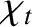(488)

which points in the same direction as the wave-vector,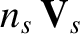, and whose magnitude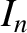is the refractive index (i.e., the ratio of the velocity of light in vacuum to the phase-velocity). Note thatshould not be confused with the particle density. Equation (458) can be rewritten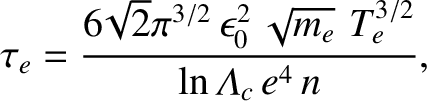(489)

We may, without loss of generality, assume that the equilibrium magnetic field is directed along the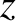-axis, and that the wave-vector,, lies in the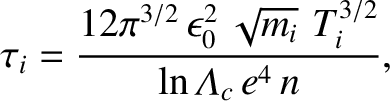-plane. Let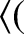be the angle subtended betweenand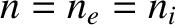. The eigenmode equation (489) can be written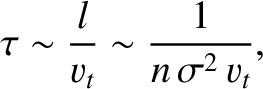(490)

The condition for a nontrivial solution is that the determinant of the square matrix be zero. With the help of the identity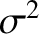(491)

we find that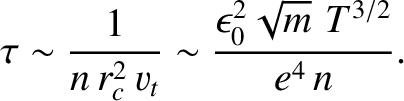(492)

where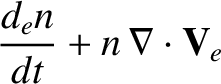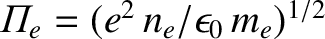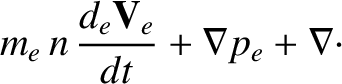(493)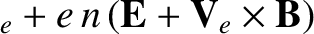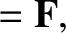(494)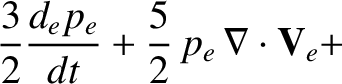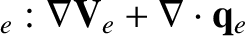(495)

The dispersion relation (492) is evidently a quadratic in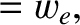, with two roots. The solution can be written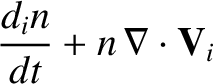(496)

where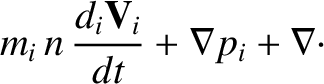(497)

Note that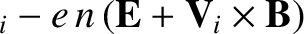. It follows thatis always real, which implies thatis either purely real or purely imaginary. In other words, the cold-plasma dispersion relation describes waves which either propagate without evanescense, or decay without spatial oscillation. The two roots of opposite sign for, corresponding to a particular root for, simply describe waves of the same type propagating, or decaying, in opposite directions.

The dispersion relation (492) can also be written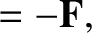(498)

For the special case of wave propagation parallel to the magnetic field (i.e.,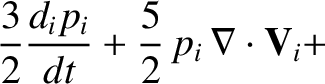), the above expression reduces to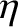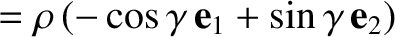(499)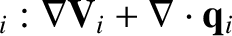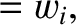(500)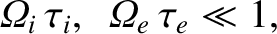(501)

Likewise, for the special case of propagation perpendicular to the field (i.e.,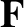), Eq. (498) yields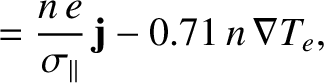(502)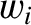(503)Next: Polarization Up: Waves in Cold Plasmas Previous: Cold-Plasma Dielectric Permittivity
Richard Fitzpatrick 2011-03-31